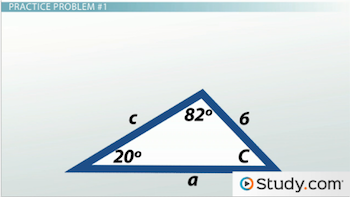# Law of Sines: Definition and Application

An error occurred trying to load this video.

Try refreshing the page, or contact customer support.

Coming up next: Law of Cosines: Definition and Application

### You're on a roll. Keep up the good work!

Replay
Your next lesson will play in 10 seconds
• 0:07 Conquering Triangles
• 1:17 Law of Sines
• 2:29 Practice Problem #1
• 3:40 Practice Problem #2
• 4:35 Practice Problem #3
• 5:27 Lesson Summary
Save Save

Want to watch this again later?

Timeline
Autoplay
Autoplay
Speed Speed

#### Recommended Lessons and Courses for You

Lesson Transcript
Instructor: Jeff Calareso

Jeff teaches high school English, math and other subjects. He has a master's degree in writing and literature.

Don't get stuck trying to find missing sides or angles in a triangle. Use the Law of Sines to rescue you from any perilous triangle in which you have just a few pieces of information.

## Conquering Triangles

Triangles aren't so scary, right? I guess it depends on context. The triangles of cheese that a sandwich shop uses are a welcome sight. The triangle on a pool table can be good or bad, depending on your pool shark-um-ness. And then there's the mysterious Bermuda Triangle, which I guess is kind of scary.

But right triangles are your friends.

If you see this one, where you know two sides and want the third, what do you do? Pythagorean Theorem! This tells us that the sum of the squares of the two legs equals the square of the hypotenuse, or a2 + b2 = c2.

And what about this one? You know this angle and the hypotenuse and need this side. Just use 'SOH CAH TOA', our awesome acronym to remember sine, cosine, and tangent. The SOH means that the sine of an angle equals the side opposite the angle over the triangle's hypotenuse.

This is the opposite side from the angle, so we say sine 30 equals the opposite over the hypotenuse, or x over 6. No problem!

## Law of Sines

Unfortunately, we don't always have right triangles. Sometimes we have wrong triangles.

Ok, a triangle like this isn't wrong. And, in fact, we can still use sine to help us. And not just 'SOH CAH TOA', but something called the Law of Sines. This law has nothing to do with where you can and can't post signs. No, it's a super-helpful tool for tackling any triangle.

It goes like this: a/sin A = b/sin B = c/sin C. With our triangle, the capital A, B and C are the angles. And the lowercase a, b and c are the lengths of these sides opposite their respective angles.

With the Law of Sines, we're comparing ratios. The ratios of the sides to the sines are equal to each other.

Again, this works with any triangle. And you'll never need to use all three sides and angles. You only need two. So if you know two angles and one side, you can use the Law of Sines to find the missing side.

Likewise, if you know two sides and an angle, you can use the Law of Sines to find the missing angle, but we're just going to focus on finding a missing side here.

## Practice Problem #1

Let's practice that first type of problem, when we know two sides and an angle. Here's a triangle.We're told that angle A is 82 degrees and angle B is 20 degrees. We also know that side b is 6 units long. We can use the Law of Sines to find the length of side a.

Remember the law? It's a/sin A = b/sin B = c/sin C. We don't need that last part. Let's just plug in what we know. So it's a/sin 82 = 6/sin 20. The sine of 82 is .99, or almost 1. The sine of 20 is about .34. Let's cross multiply. 1 times 6 is 6. a times .34 is .34a. 6 divided by .34 is about 18. So side a is about 18 units long.

To unlock this lesson you must be a Study.com Member.

### Register to view this lesson

Are you a student or a teacher?

#### See for yourself why 30 million people use Study.com

##### Become a Study.com member and start learning now.
Back
What teachers are saying about Study.com

### Earning College Credit

Did you know… We have over 200 college courses that prepare you to earn credit by exam that is accepted by over 1,500 colleges and universities. You can test out of the first two years of college and save thousands off your degree. Anyone can earn credit-by-exam regardless of age or education level.